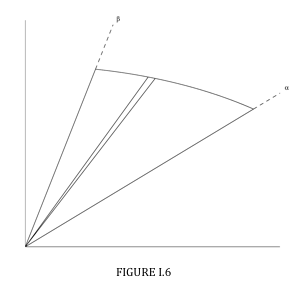$$\require{cancel}$$

1.3: Plane Areas

•• Contributed by Jeremy Tatum
• Emeritus Professor (Physics & Astronomy) at University of Victoria

Plane areas in which the equation is given in $$x-y$$ coordinatesWe have a curve $$y = y(x)$$ (Figure I.3) and we wish to find the position of the centroid of the area under the curve between $$x = a$$ and $$x = b$$. We consider an elemental slice of width $$\delta x$$ at a distance $$x$$ from the $$y$$ axis. Its area is $$y \delta x$$, and so the total area is

$A = \int_a^b ydx \label{eq:1.3.1}$

The first moment of area of the slice with respect to the $$y$$ axis is $$x y \delta x$$, and so the first moment of the entire area is $$\int_a^b xydx$$.

Therefore

$\overline{x} = \frac{ \int_a^b xydyx }{\int_a^b ydyx} = \frac{\int_a^b xydyx}{A} \\label{eq:1.3.2}$

For $$\overline{y}$$ we notice that the distance of the centroid of the slice from the $$x$$ axis is $$\frac{1}{2}y$$ , and therefore the first moment of the area about the $$x$$ axis is $$\frac{1}{2} y.y \delta x$$ .

Therefore

$\overline{y} = \frac{ \int_a^b y^2dx }{2A} \label{eq:1.3.3}$

Example $$\PageIndex{1}$$

Consider a semicircular lamina, $$x^{2} + y^{2} = a^{2}$$ , see Figure I.4:We are dealing with the parts both above and below the $$x$$ axis, so the area of the semicircle is $$2\int_0^a ydx$$ and the first moment of area is $$2\int_0^a xydx$$.

You should find $$\overline{x} = 4a/ (3 \pi) = 0.4244a$$.

Now consider the lamina $$x^{2} + y^{2} = a^{2}$$ , $$y >0$$ (Figure I.5):The area of the elemental slice this time is $$y \delta x$$ (not $$2 y \delta x$$ ), and the integration limits are from $$-a$$ to $$+a$$. To find $$\overline{y}$$, use Equation $$\ref{eq:1.3.3}$$, and you should get $$y = 0.4244a$$ .We consider an elemental triangular sector (Figure I.6) between $$\theta$$ and $$\theta + \delta \theta$$ . The "height" of the triangle is r and the "base" is $$r \delta \theta$$. The area of the triangle is $$\frac{1}{2} r^{2} \delta \theta$$.

Therefore the whole area =

$\frac{1}{2} \int_ \alpha ^ \beta r^{2}d \theta \label{eq:1.3.4}$

The horizontal distance of the centroid of the elemental sector from the origin (more correctly, from the "pole" of the polar coordinate system) is $$\frac{2}{3} r \cos \theta$$ . The first moment of area of the sector with respect to the $$y$$ axis is

$$\frac{2}{3}r\cos \theta \times \frac{1}{2} r^{2} \delta \theta = \frac{1}{3}r^{3} \cos \theta \delta \theta$$

so the first moment of area of the entire figure between $$\theta = \alpha$$ and $$\theta = \beta$$ is

$$\frac{1}{3} \int_ \alpha ^ \beta r^3 \cos \theta d \theta$$

Therefore

$\overline{x} = \frac{2 \int_ \alpha ^ \beta r^{3}\cos \theta d \theta }{3\int_ \alpha ^ \beta r^{2} d \theta} \label{eq:1.3.5}$

Similarly

$\overline{x} = \frac{2 \int_ \alpha ^ \beta r^{3}\sin \theta d \theta }{3\int_ \alpha ^ \beta r^{2} d \theta} \label{eq:1.3.6}$

Example $$\PageIndex{2}$$

Consider the semicircle $$r = a$$, $$\theta = \frac{- \pi}{2}$$ to $$\frac{+ \pi}{2}$$

$\overline{x} = \frac{2a \int_{- \pi /2}^{+ \pi /2} \cos \theta d \theta }{3 \int_{- \pi /2}^{+ \pi /2} d \theta } = \frac{2a}{3 \pi } \int_{- \pi /2}^{+ \pi /2} \cos \theta d \theta = \frac{4a}{3 \pi} \label{eq:1.3.7}$

The reader should now try to find the position of the centroid of a circular sector (slice of pizza!) of angle $$2\alpha$$ . The integration limits will be $$- \alpha$$ to $$+ \alpha$$.

When you arrive at a formula (which you should keep in a notebook for future reference), check that it goes to $$\frac{4 \alpha}{ 3 \pi}$$ if $$\alpha = \frac{ \pi}{2}$$, and to $$\frac{2 \pi}{3}$$ if $$\alpha = 0$$.